# Capacitor Charging Equation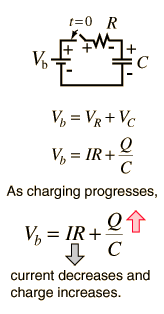The transient behavior of a circuit with a battery, a resistor and a capacitor is governed by Ohm's law, the voltage law and the definition of capacitance. Development of the capacitor charging relationship requires calculus methods and involves a differential equation. For continuously varying charge the current is defined by a derivative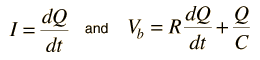This kind of differential equation has a general solution of the form: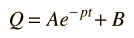and the detailed solution is formed by substitution of the general solution and forcing it to fit the boundary conditions of this problem. The result is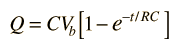Charging capacitor Capacitor discharge
Index

DC Circuits

Capacitor Concepts

 HyperPhysics***** Electricity and Magnetism R Nave
Go Back

# Capacitor Discharge Calculation

For circuit parameters:
 R = Ω, V0 = V

 C = μF, RC = s = time constant.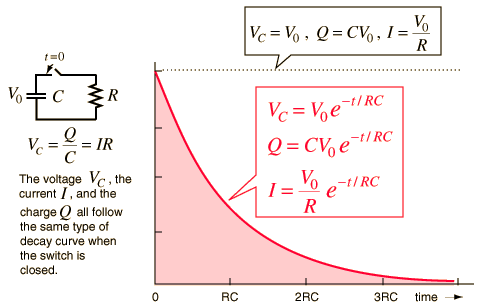This circuit will have a maximum current of Imax = A
just after the switch is closed.
 The charge will start at its maximum value Qmax= μC.
 At time t = s= RC
 the current is = Imax = A,
 the capacitor voltage is = V0 = V,
 and the charge on the capacitor is = Qmax = μC

 Capacitor discharge derivation Capacitor charging discussion
Index

DC Circuits

Capacitor Concepts

 HyperPhysics***** Electricity and Magnetism R Nave
Go Back